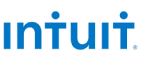New update is available. Click here to update.
Topics

# Matrix Median

Moderate0/80
Average time to solve is 15m+1 more companies

## Problem statement

You have been given a matrix of ‘N’ rows and ‘M’ columns filled up with integers where every row is sorted in non-decreasing order. Your task is to find the overall median of the matrix i.e if all elements of the matrix are written in a single line, then you need to return the median of that linear array.

The median of a finite list of numbers is the "middle" number when those numbers are listed in order from smallest to greatest. If there is an odd number of observations, the middle one is picked. For example, consider the list of numbers [1, 3, 3, 6, 7, 8, 9]. This list contains seven numbers. The median is the fourth of them, which is 6.

Detailed explanation ( Input/output format, Notes, Images )
Constraints:
``````1 <= 'T' <= 50
1 <= 'N' , 'M' <= 100
1 <= 'MATRIX'['I']['J'] <= 10 ^ 5
'N' * 'M' is always an odd number.

Where 'MATRIX'['I']['J']  denotes the value at ('I', 'J')th cell in the matrix.

Time limit: 1 sec
``````
Sample Input 1:
``````2
1 3
1 2 3
3 3
2 6 9
1 5 11
3 7 8
``````
Sample Output 1:
``````2
6
``````
Explanation of sample input 1:
``````In the first test case, the overall median of the matrix is 2.

In the second test case, the overall median of the matrix is 6.
``````
Sample Input 2:
``````2
3 3
2 6 8
1 4 7
6 8 9
3 5
1 2 6 6 10
2 4 4 5 7
2 5 5 6 6
``````
Sample Output 2:
``````2
5
``````
Explanation for sample input 2:
``````In the first test case, the overall median of the matrix is 2.

In the second test case, the overall median of the matrix is 5.
``````Console